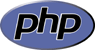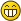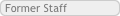Alternative to 'else if' statement using a loop?Greg Winstonis not my name.Posts: 509 Threads: 153 Joined: Jul 2013 Reputation: 3 2015-01-21, 11:19 PM (This post was last modified: 2015-01-21, 11:20 PM by Greg Winston.) Here is an example of the code I have: ``````\$x = 7; // This number could be any number between 1 and infinity. (It will never be infinity, usually the number will be between 1 & 100). if (\$x === 1) { \$number = 1; } else if (\$x === 2) { \$number = 2; } else if (\$x === 3) { \$number = 3; } else if (\$x === 4) { \$number = 4; } else if (\$x === 5) { \$number = 5; } else if (\$x === 6) { \$number = 6; } else if (\$x === 7) { \$number = 7; } else if (\$x === 8) { \$number = 8; } else if (\$x === 9) { \$number = 9; } else if (\$x === 10) { \$number = 10; } else if (\$x === 11) { \$number = 11; } else if (\$x === 12) { \$number = 12; //} else if (etc...to infinity, until it finds what \$x is equal to)`````` Is there a loop that I could use, to achieve the same functionality, because it would need to loop from 1 until it finds a number. It will never loop infinite times, because it will always find a number, I just don't know what that number is. Which loop could I use?Ad BakkerAlways learningPosts: 1,062 Threads: 24 Joined: Jun 2011 Reputation: 68 2015-01-22, 08:59 AM (This post was last modified: 2015-01-22, 09:01 AM by Ad Bakker.) ``````\$x = 7; \$number = 1; while(\$x != \$number) {    \$number++; } ``````Something like this? But isn't this the same as \$number = \$x ?Regards, Ad Bakker (NL)dragonexpertApproved DeveloperPosts: 4,041 Threads: 127 Joined: Dec 2009 Reputation: 236 2015-01-22, 01:33 PM Consider using a Do While Loop. Something else you can do is a for loop and test the condition in it. If the condition is true use the keyword break.Greg Winstonis not my name.Posts: 509 Threads: 153 Joined: Jul 2013 Reputation: 3 2015-01-23, 04:11 AM (This post was last modified: 2015-01-23, 04:13 AM by Greg Winston.) (2015-01-22, 08:59 AM)Ad Bakker Wrote: ``````\$x = 7; \$number = 1; while(\$x != \$number) {    \$number++; } ``````Something like this? But isn't this the same as \$number = \$x ?Close, but how would I make it show the value of \$number after it loops? Right now it just outputs 123456, and then the loop ends at 7.Ad BakkerAlways learningPosts: 1,062 Threads: 24 Joined: Jun 2011 Reputation: 68 2015-01-23, 07:34 AM That is weird. I ran the following test: `````` `````` and it displayed "number = 7". Regards, Ad Bakker (NL)Greg Winstonis not my name.Posts: 509 Threads: 153 Joined: Jul 2013 Reputation: 3 2015-01-23, 09:55 PM Your code works. Thank you very much. « Next Oldest | Next Newest »

Forum Jump:

Users browsing this thread: 1 Guest(s)